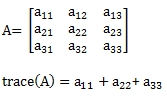# SQL Server matrix trace

MTRACE

Updated: 30 September 2013

Use MTRACE to calculate the trace of a de-normalized matrix. The trace of a matrix (A) is the sum of the diagonal elements. For example:Syntax
SELECT [wctMath].[wct].[MTRACE](
<@Matrix_TableName, nvarchar(max),>
,<@ColumnNames, nvarchar(max),>
,<@GroupedColumnName, nvarchar(4000),>
,<@GroupedColumnValue, sql_variant,>)
Arguments
@Matrix_ TableName
the name, as text, of the table or view that contains the matrix values. @Matrix_ TableName cannot reference a table variable or a common table expression.
@Matrix_ ColumnNames
the name, as text, of the columns in the table or view specified by @Matrix_TableName that contains the matrix values to be used in the calculation. Data returned from the @Matrix_ColumnNames must be of the type float or of a type that implicitly converts to float.
@Matrix_GroupedColumnName
the name, as text, of the column in the table or view specified by @Matrix_TableName which will be used for grouping the results.
@Matrix_GroupedColumnValue
the column value to do the grouping on.
Return Types
float
Remarks
·         Use the MTRACE_q function for more complex queries.
·         Use MTRACEN for a table in 3rd normal form.
·         If the matrix is not square then an error will be returned.
·         If the matrix diagonal contains NULL, then NULL will be returned.
·         The function returns an error if the matrix diagonal contains a non-numeric value.
Examples
In this example, we calculate the trace from a temporary table containing one matrix.
SET NOCOUNT ON

SELECT *
INTO #c
FROM (VALUES
(-78,-35,98,-65,49),
(48,-53,-1,-18,-12),
(35,-70,-77,44,73),
(37,-75,-13,-55,97),
(-56,36,84,-34,52)
)n(x1,x2,x3,x4,x5)

SELECT wct.MTRACE('#c','x1,x2,x3,x4,x5','',NULL) as TRACE

DROP TABLE #c
This produces the following result.
TRACE
----------------------
-211

Using the same data, we can calculate the trace directly from the derived table.
SELECT wct.MTRACE('(VALUES
(-78,-35,98,-65,49),
(48,-53,-1,-18,-12),
(35,-70,-77,44,73),
(37,-75,-13,-55,97),
(-56,36,84,-34,52)
)n(x1,x2,x3,x4,x5)','x1,x2,x3,x4,x5','',NULL) as TRACE

This produces the following result.
TRACE
----------------------
-211

Let's put several matrices into a table and calculate the trace for each.
SET NOCOUNT ON

CREATE TABLE #c(
Matrix      int,
rn          int,
x1          float,
x2          float,
x3          float,
PRIMARY KEY (Matrix, rn)
)

INSERT INTO #c VALUES (500,1,88,32,-19)
INSERT INTO #c VALUES (500,2,-14,-7,-87)
INSERT INTO #c VALUES (500,3,-99,-43,30)
INSERT INTO #c VALUES (501,1,-10,-69,25)
INSERT INTO #c VALUES (501,2,-94,83,-40)
INSERT INTO #c VALUES (501,3,0,-1,20)
INSERT INTO #c VALUES (502,1,-73,-55,1)
INSERT INTO #c VALUES (502,2,-84,-41,-92)
INSERT INTO #c VALUES (502,3,-70,-69,-70)
INSERT INTO #c VALUES (503,1,-41,-90,86)
INSERT INTO #c VALUES (503,2,-90,88,24)
INSERT INTO #c VALUES (503,3,52,60,-37)

SELECT Matrix
,wct.MTRACE('#c','x1,x2,x3','Matrix',n.Matrix) as Trace
FROM (SELECT DISTINCT Matrix FROM #c)n

DROP TABLE #c

This produces the following result.
Matrix                  Trace
----------- ----------------------
500                    111
501                     93
502                   -184
503                     10

### SupportCopyright 2008-2021 Westclintech LLC         Privacy Policy        Terms of Service